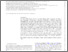# Stellar Property Statistics of Massive Halos from Cosmological Hydrodynamics Simulations: Common Kernel Shapes

Anbajagane, D, Evrard, AE, Farahi, A, Barnes, DJ, Dolag, K, McCarthy, IG, Nelson, D and Pillepich, A (2020) Stellar Property Statistics of Massive Halos from Cosmological Hydrodynamics Simulations: Common Kernel Shapes. Monthly Notices of the Royal Astronomical Society, 495 (1). pp. 686-704. ISSN 0035-8711Preview
Text
paper2.pdf - Published Version

We study stellar property statistics, including satellite galaxy occupation, of massive halo populations realized by three cosmological hydrodynamics simulations: BAHAMAS + MACSIS, TNG300 of the IllustrisTNG suite, and Magneticum Pathfinder. The simulations incorporate independent sub-grid methods for astrophysical processes with spatial resolutions ranging from $1.5$ to $6$ kpc, and each generates samples of $1000$ or more halos with $M_{\rm halo}> 10^{13.5} M_{\odot}$ at redshift $z=0$. Applying localized, linear regression (LLR), we extract halo mass-conditioned statistics (normalizations, slopes, and intrinsic covariance) for a three-element stellar property vector consisting of: i) $N_{sat}$, the number of satellite galaxies with stellar mass, $M_{\star, \rm sat} > 10^{10} M_{\odot}$ within radius $R_{200c}$ of the halo; ii) $M_{\star,\rm tot}$, the total stellar mass within that radius, and; iii) $M_{\star,\rm BCG}$, the gravitationally-bound stellar mass of the central galaxy within a $100 \, \rm kpc$ radius. Scaling parameters for the three properties with halo mass show mild differences among the simulations, in part due to numerical resolution, but there is qualitative agreement on property correlations, with halos having smaller than average central galaxies tending to also have smaller total stellar mass and a larger number of satellite galaxies. Marginalizing over total halo mass, we find the satellite galaxy kernel, $p(\ln N_{sat}\,|\,M_{\rm halo},z)$ to be consistently skewed left, with skewness parameter $\gamma = -0.91 \pm 0.02$, while that of $\ln M_{\star,\rm tot}$ is closer to log-normal, in all three simulations. The highest resolution simulations find $\gamma \simeq -0.8$ for the $z=0$ shape of $p(\ln M_{\star,\rm BCG}\,|\,M_{\rm halo},z)$ and also that the fractional scatter in total stellar mass is below $10\%$ in halos more massive than $10^{14.3} M_{\odot}$.View Item